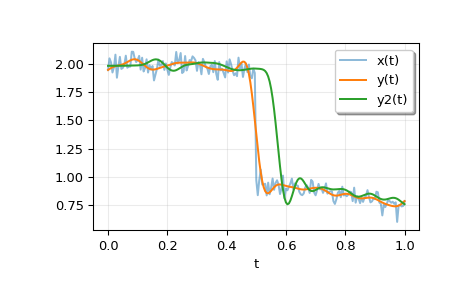# scipy.signal.sosfiltfilt¶

scipy.signal.sosfiltfilt(sos, x, axis=-1, padtype='odd', padlen=None)[source]

A forward-backward digital filter using cascaded second-order sections.

See filtfilt for more complete information about this method.

Parameters
sosarray_like

Array of second-order filter coefficients, must have shape (n_sections, 6). Each row corresponds to a second-order section, with the first three columns providing the numerator coefficients and the last three providing the denominator coefficients.

xarray_like

The array of data to be filtered.

axisint, optional

The axis of x to which the filter is applied. Default is -1.

Must be ‘odd’, ‘even’, ‘constant’, or None. This determines the type of extension to use for the padded signal to which the filter is applied. If padtype is None, no padding is used. The default is ‘odd’.

The number of elements by which to extend x at both ends of axis before applying the filter. This value must be less than x.shape[axis] - 1. padlen=0 implies no padding. The default value is:

3 * (2 * len(sos) + 1 - min((sos[:, 2] == 0).sum(),
(sos[:, 5] == 0).sum()))


The extra subtraction at the end attempts to compensate for poles and zeros at the origin (e.g. for odd-order filters) to yield equivalent estimates of padlen to those of filtfilt for second-order section filters built with scipy.signal functions.

Returns
yndarray

The filtered output with the same shape as x.

Notes

New in version 0.18.0.

Examples

>>> from scipy.signal import sosfiltfilt, butter
>>> import matplotlib.pyplot as plt


Create an interesting signal to filter.

>>> n = 201
>>> t = np.linspace(0, 1, n)
>>> np.random.seed(123)
>>> x = 1 + (t < 0.5) - 0.25*t**2 + 0.05*np.random.randn(n)


Create a lowpass Butterworth filter, and use it to filter x.

>>> sos = butter(4, 0.125, output='sos')
>>> y = sosfiltfilt(sos, x)


For comparison, apply an 8th order filter using sosfilt. The filter is initialized using the mean of the first four values of x.

>>> from scipy.signal import sosfilt, sosfilt_zi
>>> sos8 = butter(8, 0.125, output='sos')
>>> zi = x[:4].mean() * sosfilt_zi(sos8)
>>> y2, zo = sosfilt(sos8, x, zi=zi)


Plot the results. Note that the phase of y matches the input, while y2 has a significant phase delay.

>>> plt.plot(t, x, alpha=0.5, label='x(t)')
>>> plt.plot(t, y, label='y(t)')
>>> plt.plot(t, y2, label='y2(t)')